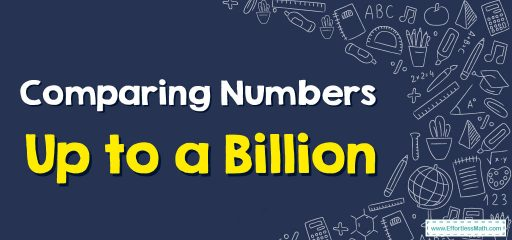# How to Compare Numbers Up to a Billion: A Step-by-Step Guide

For comparing 2 numbers, firstly count the number of digits. If a number has an unequal number of digits, then the number that has more digits is larger. If a number has the same number of digits, then you compare these numbers digit by digit beginning with the digit which is in the highest place value.## A step-by-step guide to comparing numbers up to a billion

Here’s a step-by-step guide on how to compare numbers up to a billion:

### Step 1: Understand Number Place Values

The first step is understanding how place values work. Each digit in a number has a place value, depending on its position. From right to left:

• The first place is the ones place.
• The second place is the tens place.
• The third place is the hundreds place.
• The fourth place is the thousands place.
• The fifth place is the ten thousands place.
• The sixth place is the hundred thousands place.
• The seventh place is the millions place.
• The eighth place is the ten millions place.
• The ninth place is the hundred millions place.
• The tenth place is the billions place.

### Step 2: Count the Number of Digits

When comparing numbers, first count how many digits each number has. The number with more digits is larger. For example, 10,000 (5 digits) is larger than 9,999 (4 digits).

### Step 3: Compare from Highest Place Value

If the numbers have the same number of digits, start comparing from the left-most digit (the highest place value). The number with the larger digit in the left-most place is larger. For example, between 700,000 and 600,000, 700,000 is larger because 7 is larger than 6.

### Step 4: Continue to the Next Place Value

If the numbers in the highest place value are the same, move to the next place value. For example, with 560,000 and 580,000, the first digits are the same, so you move to the next place. 8 is larger than 6, so 580,000 is larger.

### Step 5: Repeat Until a Difference is Found

Continue this process until you find a place where the numbers are different. For example, between 543,210,000 and 543,203,000, you have to go to the hundred thousands place to find that the first number is larger.

### Step 6: Practice

The best way to understand this is to practice. Try comparing these pairs of numbers:

• 1,000,000,000 and 999,999,999
• 123,456,789 and 123,456,788
• 500,000,000 and 500,000,001

Remember, the key to comparing numbers is understanding place values and starting from the largest place value when comparing.

### Example:

Compare number 3,941 with number 3,986. Which number is greater?

Solution: See that both these numbers have digits. Let’s write down the numbers inside a place value chart.

See that the thousands digit in both these numbers is the same. Additionally, both the hundreds digits are the same.

Thus, you compare the tens digits. The tens digit in 3941 is 4 and the tens in 3986 is 8. So, 3941 is less than 3986.

Hence, 3941<3986.

### What people say about "How to Compare Numbers Up to a Billion: A Step-by-Step Guide - Effortless Math: We Help Students Learn to LOVE Mathematics"?

No one replied yet.

X
30% OFF

Limited time only!

Save Over 30%

SAVE $5 It was$16.99 now it is \$11.99